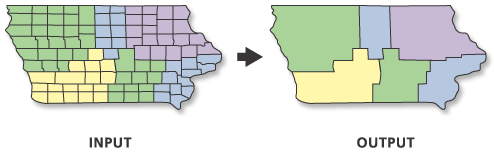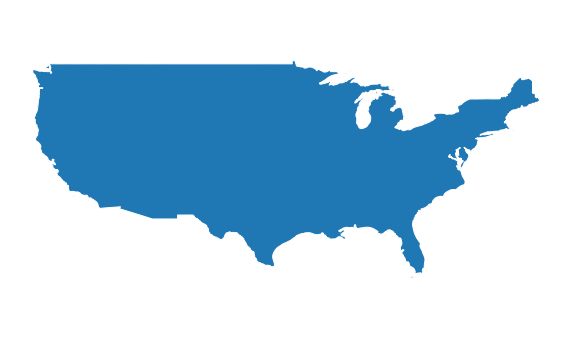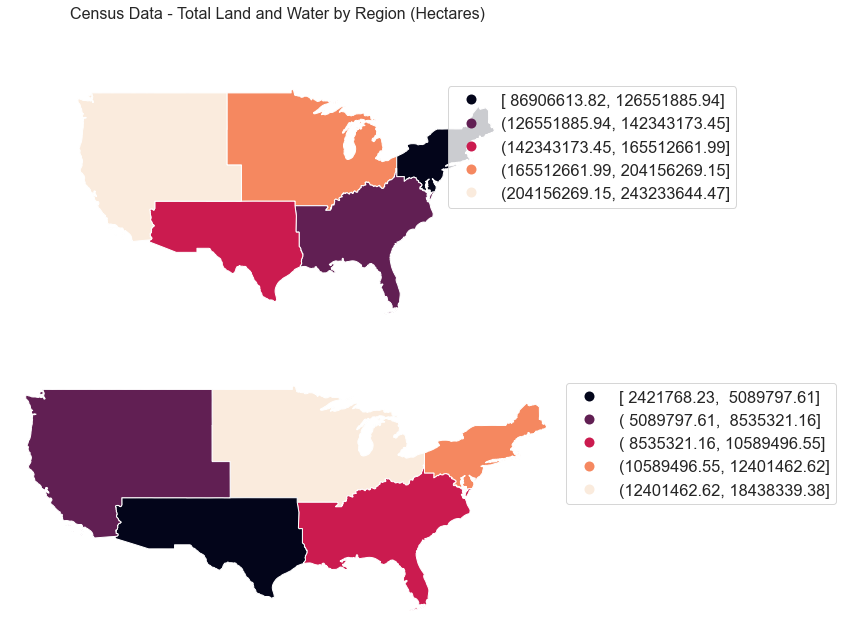# Lesson 4. How to Dissolve Polygons Using Geopandas: GIS in Python

## Learning Objectives

• Dissolve polygons based upon an attribute using geopandas in Python.

### Import Packages and Set Working Directory

# Import libraries
import os
import numpy as np
import matplotlib.pyplot as plt
import matplotlib.lines as mlines
from matplotlib.colors import ListedColormap
import seaborn as sns
from shapely.geometry import box
import geopandas as gpd
import earthpy as et

sns.set(font_scale=1.5)
sns.set_style("white")

# Set working dir & get data
data = et.data.get_data('spatial-vector-lidar')
os.chdir(os.path.join(et.io.HOME, 'earth-analytics'))


### Import Data

To begin, import the following foud shapefiles using geopandas.

# Define base path as it is repeated below
base_path = os.path.join("data", "spatial-vector-lidar")

# Define file paths
country_boundary_path = os.path.join(base_path, "usa",
"usa-boundary-dissolved.shp")

state_boundary_path = os.path.join(base_path, "usa",
"usa-states-census-2014.shp")

pop_places_path = os.path.join(base_path, "global", "ne_110m_populated_places_simple",
"ne_110m_populated_places_simple.shp")

# Import the data


## Dissolve Polygons Based On an Attribute with Geopandas

Next, you will learn how to dissolve polygon data. Dissolving polygons entails combining polygons based upon a unique attribute value and removing the interior geometry.When you dissolve polygons you remove interior boundaries of a set of polygons with the same attribute value and create one new "merged" or combined polygon for each attribute value. In the case above US states are dissolved to regions in the United States. Source: ESRI

Below you will dissolve the US states polygons by the region that each state is in. When you dissolve, you will create a new set polygons - one for each regions in the United States.

To begin, explore your data. Using .geom_type you can see that you have a mix of single and multi polygons in your data. Sometimes multi-polygons can cause problems when processing. It’s always good to check your geometry before you begin to better know what you are working with.

state_boundary_us.head()

006017797780400000US0606CACalifornia0040348382318120483271881WestMULTIPOLYGON Z (((-118.59397 33.46720 0.00000,...
111017023820400000US1111DCDistrict of Columbia0015835057818633500NortheastPOLYGON Z ((-77.11976 38.93434 0.00000, -77.04...
212002944780400000US1212FLFlorida0013890320085531407883551SoutheastMULTIPOLYGON Z (((-81.81169 24.56874 0.00000, ...
313017053170400000US1313GAGeorgia001489635033994947080103SoutheastPOLYGON Z ((-85.60516 34.98468 0.00000, -85.47...
416017797830400000US1616IDIdaho002140454255492397728105WestPOLYGON Z ((-117.24303 44.39097 0.00000, -117....
state_boundary_us.geom_type.head()

0    MultiPolygon
1         Polygon
2    MultiPolygon
3         Polygon
4         Polygon
dtype: object


Next, select the columns that you with to use for the dissolve and keep. In this case we want to retain the:

• geometry

columns.

state_boundary = state_boundary_us[['LSAD', 'geometry']]

# View the resulting geodataframe
cont_usa

geometry
00MULTIPOLYGON Z (((-81.81169 24.56874 0.00000, ...

And finally, plot the data. Note that when you dissolve, the column used to perform the dissolve becomes an index for the resultant geodataframe. Thus you will have to use the reset_index() method when you plot, to access the region “column”.

So this will work:

us_regions.reset_index().plot(column = 'region', ax=ax)

This will return an error as region is no longer a column, it is an index! us_regions.plot(column = 'region', ax=ax)

cont_usa.reset_index()

000MULTIPOLYGON Z (((-81.81169 24.56874 0.00000, ...
# Plot the data
fig, ax = plt.subplots(figsize=(10, 6))
ax=ax)
ax.set_axis_off()
plt.axis('equal')
plt.show()The LSAD attribute value for every polygon in the data is 00. Thus when you dissolve by that attribute, you get one resulting polygon.

## Dissolve and Aggregate Data

In the example above, you dissolved the state level polygons to a region level. However, you did not aggregate or summarize the attributes associated with each polygon. Next, you will learn how to aggregate quantitative values in your attribute table when you perform a dissolve. To do this, you will add

aggfunc = 'fun-here'

to your dissolve call. You can choice a suite of different summary functions including:

• first
• last
• mean
• max

Below the data are aggregated by the ‘sum’ method. This means that the values for ALAND are added up for all of the states in a region. That summary sum value will be returned in the new dataframe.

# Select the columns that you wish to retain in the data
state_boundary = state_boundary_us[['region', 'geometry', 'ALAND', 'AWATER']]

# Then summarize the quantative columns by 'sum'
regions_agg = state_boundary.dissolve(by='region', aggfunc='sum')
regions_agg

geometryALANDAWATER
region
MidwestMULTIPOLYGON Z (((-87.80048 42.49192 0.00000, ...1943869253244184383393833
NortheastMULTIPOLYGON Z (((-76.04621 38.02553 0.00000, ...869066138232108922434345
SoutheastMULTIPOLYGON Z (((-81.81169 24.56874 0.00000, ...1364632039655103876652998
SouthwestPOLYGON Z ((-94.48587 33.63787 0.00000, -94.41...146263153099724217682268
WestMULTIPOLYGON Z (((-104.05325 41.00141 0.00000,...243233644473057568049509
# Convert area units from square meters to hectares (divide by 10,000)
regions_agg["land_ha"] = regions_agg["ALAND"] / 10000
regions_agg["water_ha"] = regions_agg["AWATER"] / 10000
regions_agg

geometryALANDAWATERland_hawater_ha
region
MidwestMULTIPOLYGON Z (((-87.80048 42.49192 0.00000, ...19438692532441843833938331.943869e+081.843834e+07
NortheastMULTIPOLYGON Z (((-76.04621 38.02553 0.00000, ...8690661382321089224343458.690661e+071.089224e+07
SoutheastMULTIPOLYGON Z (((-81.81169 24.56874 0.00000, ...13646320396551038766529981.364632e+081.038767e+07
SouthwestPOLYGON Z ((-94.48587 33.63787 0.00000, -94.41...1462631530997242176822681.462632e+082.421768e+06
WestMULTIPOLYGON Z (((-104.05325 41.00141 0.00000,...2432336444730575680495092.432336e+085.756805e+06
fig, (ax1, ax2) = plt.subplots(2, 1, figsize=(10, 10))

regions_agg.plot(column='land_ha',
legend=True,
scheme="quantiles",
ax=ax1)

regions_agg.plot(column='water_ha',
scheme="quantiles",
legend=True,
ax=ax2)

plt.suptitle('Census Data - Total Land and Water by Region (Hectares)', fontsize=16)
leg = ax1.get_legend()
leg.set_bbox_to_anchor((1.5,1))

leg = ax2.get_legend()
leg.set_bbox_to_anchor((1.5,1))
ax1.set_axis_off()
ax2.set_axis_off()

plt.axis('equal')
plt.show()In this example, you dissolved by region. There are 5 unique region values in the attributes. Thus you end up with 5 polygons. You also summarized attributes by ALAND and AWATER calculating the total value for each.